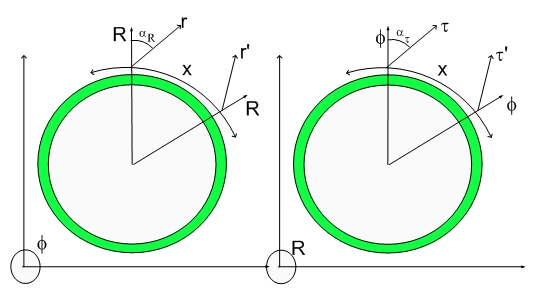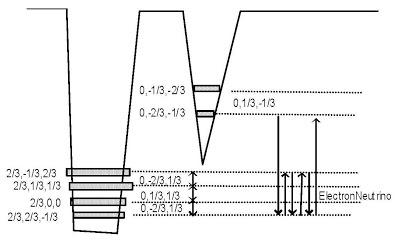# Hypergeometrical Universe

## Pion MinusPions and Neutrinos

Their coherence map is given by the four notes. Any spin half particle has always a four notes chord. I don't know anything about music, but I will make the analogy between a 180 degrees tunelling to a musical note. Since 180 degrees tunelling corresponds to 90 degrees spinning and 360 degrees corresponds to four of those events, each particle corresponds to at least four notes.The coherence is between the two Electron states. They differ just by a rotation around x. Axis are given by (0,-2/3,-1/3) and (0,-1/3,-2/3). The boldening of the first axis lenght or quantum number means that spin happens in a direction perpendicular to the x-axis and the radial axis (the fourth dimension). This is important since one has to add up half-spin associated with each chord. The neutrino chord has spin half, similarly to the neutrino particle of the standard model.

It is important to notice that the tunneling frequency between the two Electron states is different from the fundamental dilator frequency and thus the interaction among neutrinos and the rest of the Universe goes in and out of phase as the neutrinos travels, resulting in a vanishingly small interaction. This is why Neutrinos are low interacting particles. The other reason can be understood from the fact that the 4D particle is the rotation of a two dimensional ellipse (with non-zero y and z axes) around the axis perpendicular to the Radial direction and the x direction. This would result in zero 3D projection or zero 3D mass.

The mass is also a function (3D Projection) of the total displacement volume of the dilator. The neutrino having the smallest axis lenghts and being part of the electron potential well, will also have the smallest 3D mass (3D projection of the 4D displacement volume).

Notice that the transmultation notes in the Neutron Model have half-neutrino note in their composition (the note is composed of of the four coherence arrows).

The neutrino model is necessary to be able to understand the pion model. The reason for that is because a pion minus will decay into an electron and a neutrino.

## Electron-Proton Fundamental Dilator

or
RSS FeedCreate your own visitor map